# Formula - Definition with Examples

The Complete K-5 Math Learning Program Built for Your Child

• 30 Million Kids

Loved by kids and parent worldwide

• 50,000 Schools

Trusted by teachers across schools

• Comprehensive Curriculum

Aligned to Common Core

What is Formula?

The formula is a fact or a rule written with mathematical symbols. It usually connects two or more quantities with an equal to sign. When you know the value of one quantity, you can find the value of the other using the formula.

Examples of formula:

Perimeter of rectangle = 2(length + width)

If the length and width of a rectangle are ‘a’ units and ‘b’ units respectively, the formula of its perimeter is:

P = 2 (a + b)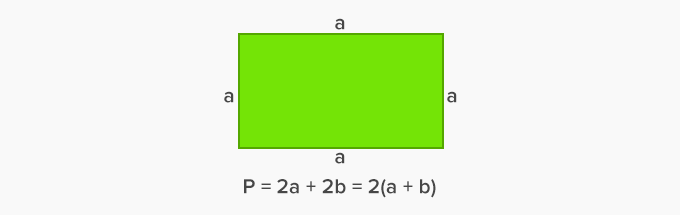Area of rectangle = length × width

If the length and width of a rectangle are ‘a’ units and ‘b’ units respectively, the formula of its area is:

P = a × b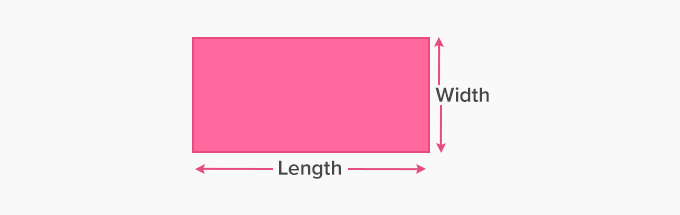Perimeter of square = 4 × side length

If the length of the side of a square is ‘a’ units, then its perimeter P is the sum of all its sides.

P = a + a + a + a

P = 4a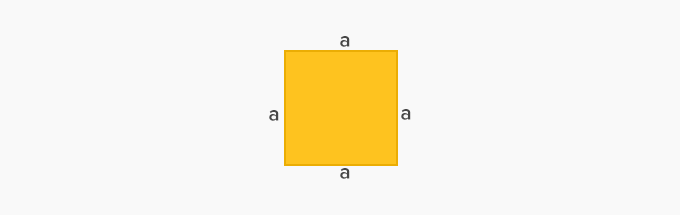Area of square = Side length × side length

If the side length of the square is a units; then its area is:

Area = a × a = a²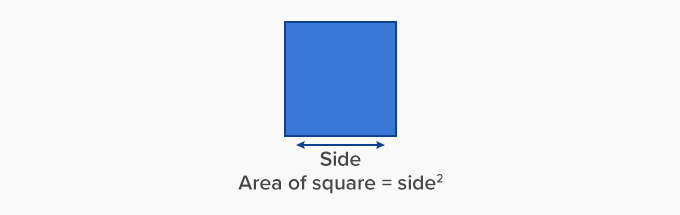Volume of cuboid = length × width × height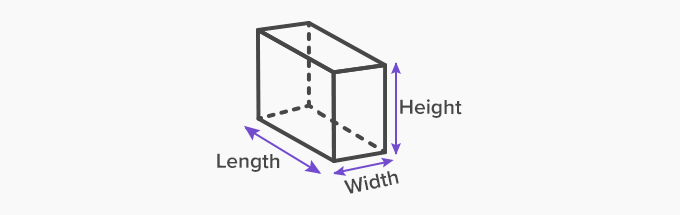Profit = Selling price - cost price

Loss = Cost price - selling price

Non examples:

2x - 3 = 6

x + y = 10

3x - 8x + 9x = 17

These are equations and not formula.

 Fun Facts The first formula was invented between 1800-1600 BC. You find formulas not just in Mathematics but in Science as well.

Won Numerous Awards & Honors